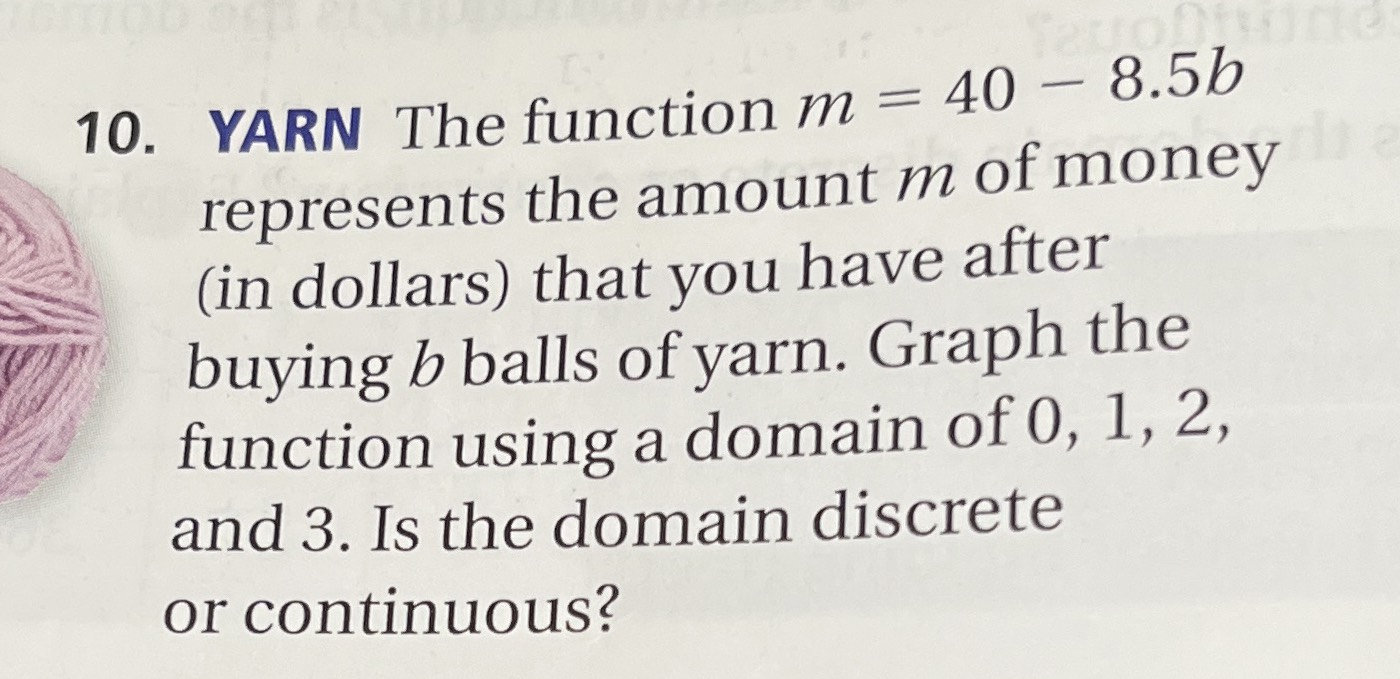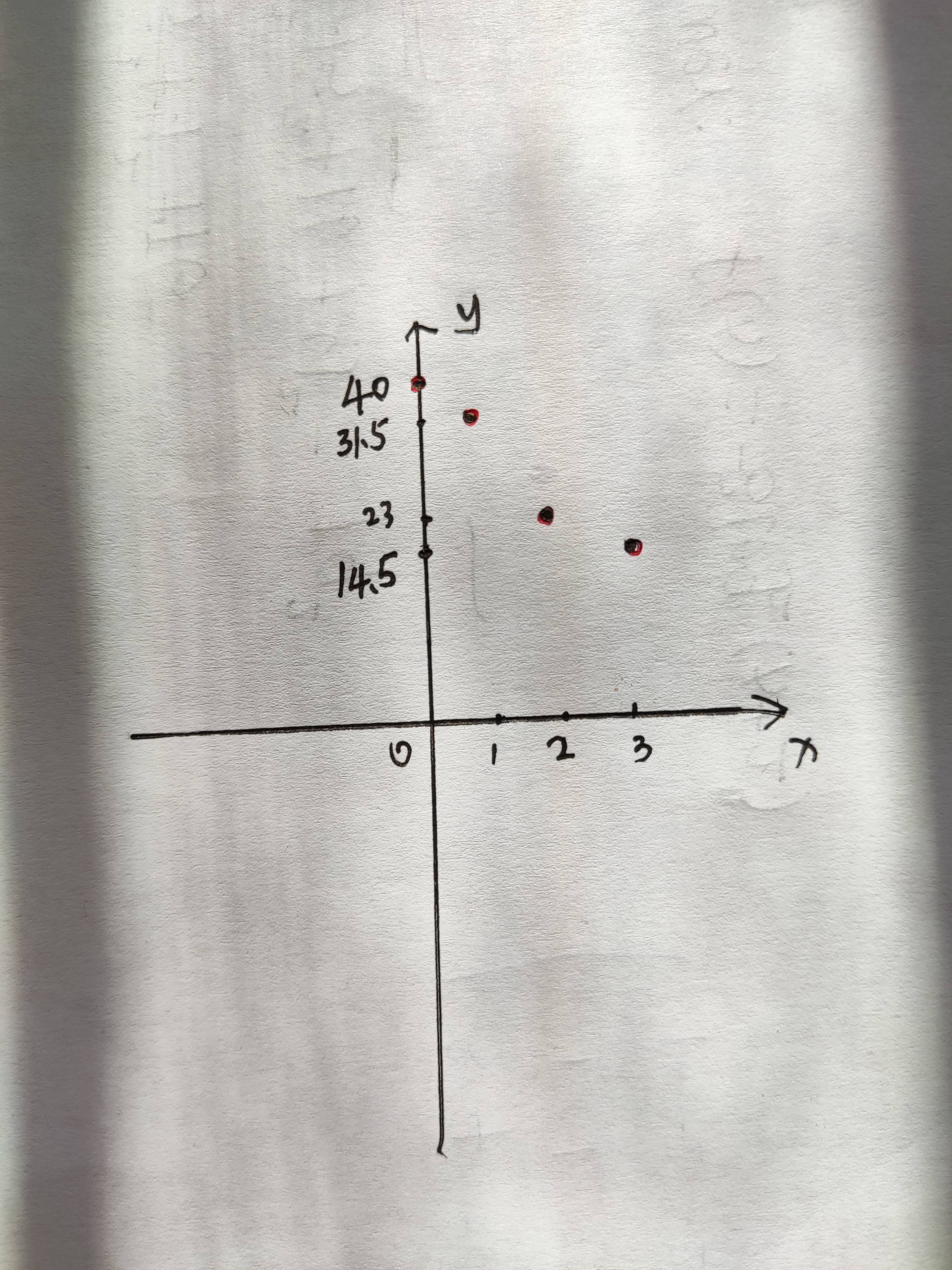### ¿Todavía tienes preguntas de matemáticas?

Pregunte a nuestros tutores expertos
Algebra
Pregunta10. YARN The function $$m = 40 - 8.5 b$$ represents the amount $$m$$ of money (in dollars) that you have after buying $$b$$ balls of yarn. Graph the function using a domain of $$0,1,2$$ , and 3. Is the domain discrete or continuous?